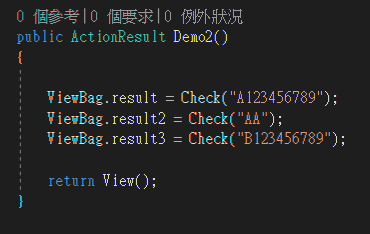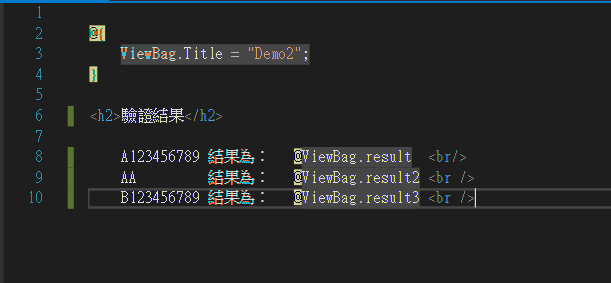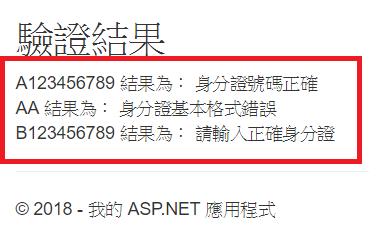#2019 iT 邦幫忙鐵人賽DAY 4
0

## 【Day 4】 在Visual Studio 2017 建立身分證驗證器

(1)英文代號以下表轉換成數字
A=10 台北市　　　J=18 新竹縣　　　S=26 高雄縣
B=11 台中市　　　K=19 苗栗縣　　　T=27 屏東縣
C=12 基隆市　　　L=20 台中縣　　　U=28 花蓮縣
D=13 台南市　　　M=21 南投縣　　　V=29 台東縣
E=14 高雄市　　　N=22 彰化縣　　 W=32 金門縣
F=15 台北縣　 O=35 新竹市　　　X=30 澎湖縣
G=16 宜蘭縣　　　P=23 雲林縣　　　Y=31 陽明山
H=17 桃園縣　　　Q=24 嘉義縣　　 Z=33 連江縣
I=34 嘉義市　 R=25 台南縣

(2)首位數字區分性別，男性為1、女性為2

130為10的倍數，正確

``````using System.Text.RegularExpressions;
``````

Step 1. 寫一個驗證 Function

``````public string Check(string id)
{
// 使用「正規表達式」檢驗格式 [A~Z] {1}個數字 [0~9] {9}個數字
var regex = new Regex("^[A-Z]{1}[0-9]{9}\$");
if (!regex.IsMatch(id))
{
//Regular Expression 驗證失敗，回傳 ID 錯誤
return "身分證基本格式錯誤";
}

//除了檢查碼外每個數字的存放空間
int[] seed = new int;

//建立字母陣列(A~Z)
//A=10 B=11 C=12 D=13 E=14 F=15 G=16 H=17 J=18 K=19 L=20 M=21 N=22
//P=23 Q=24 R=25 S=26 T=27 U=28 V=29 X=30 Y=31 W=32  Z=33 I=34 O=35
string[] charMapping = new string[] { "A", "B", "C", "D", "E", "F", "G", "H", "J", "K", "L", "M", "N", "P", "Q", "R", "S", "T", "U", "V", "X", "Y", "W", "Z", "I", "O" };
string target = id.Substring(0, 1); //取第一個英文數字
for (int index = 0; index < charMapping.Length; index++)
{
if (charMapping[index] == target)
{
index += 10;
//10進制的高位元放入存放空間   (權重*1)
seed = index / 10;

//10進制的低位元*9後放入存放空間 (權重*9)
seed = (index % 10) * 9;

break;
}
}
for (int index = 2; index < 10; index++) //(權重*8~1)
{   //將剩餘數字乘上權數後放入存放空間
seed[index] = Convert.ToInt32(id.Substring(index - 1, 1)) * (10 - index);
}
//檢查是否符合檢查規則，10減存放空間所有數字和除以10的餘數的個位數字是否等於檢查碼
//(10 - ((seed + .... + seed) % 10)) % 10 == 身分證字號的最後一碼
if ((10 - (seed.Sum() % 10)) % 10 != Convert.ToInt32(id.Substring(9, 1)))
{
return "請輸入正確身分證";
}

return "身分證號碼正確";
}
``````

Step 2 ： 在Controller創建Demo2，使用Function驗證數字

`````` public ActionResult Demo2()
{

ViewBag.result = Check("A123456789");
ViewBag.result2 = Check("AA");
ViewBag.result3 = Check("B123456789");

return View();
}
``````Step 3 : View驗證畫面

``````@{
ViewBag.Title = "Demo2";
}

<h2>驗證結果</h2>

A123456789 結果為：   @ViewBag.result  <br/>
AA         結果為：   @ViewBag.result2 <br />
B123456789 結果為：   @ViewBag.result3 <br />
``````Step 4 驗證結果